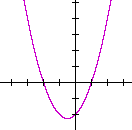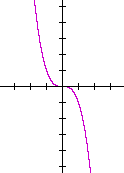Topics in

P R E C A L C U L U S

15

# REFLECTIONS

CONSIDER THE FIRST QUADRANT point (a, b), and let us reflect it about the y-axis.  It is reflected to the second quadrant point (−a, b).If we reflect (a, b) about the x-axis, then it is reflected to the fourth quadrant point (a, −b).

Finally, if we reflect (a, b) through the origin, then it is reflected to the third quadrant point (−a, −b).  The distance from the origin to (a, b) is equal to the distance from the origin to (−a, −b).

Example 1.Fig. 1 is the graph of the parabola

f(x) = x2 − 2x − 3 = (x + 1)(x − 3).

The roots −1, 3 are the x-intercepts.

Fig. 2 is its reflection about the x-axis.  Every point that was above the x-axis gets reflected to below the x-axis.  And every point below the x-axis gets reflected above the x-axis.  Only the roots, −1 and 3, are invariant.

Again, Fig. 1 is  y = f(x).  Its reflection about the x-axis is  y = −f(x).  Every y-value there is the negative of the original f(x).Fig. 3 is the reflection of  Fig. 1 about the y-axis.  Every point that was to the right of the origin gets reflected to the left.  And every point that was on the left gets reflected to the right.  Every  x  becomes  −x. Only the y-intercept is invariant.  The equation of the reflection of f(x) about the y-axis is  y = f(−x).

The argument x of f(x) is replaced by −x.  See Problem 1c) below.

If y = f(x), then

y = f(−x) is its reflection about the y-axis,

y = −f(x) is its reflection about the x-axis.

Problem 1.   Let f(x) = x2 + x − 2.

a)  Sketch the graph of f(x).

To see the answer, pass your mouse over the colored area.
To cover the answer again, click "Refresh" ("Reload").

x2 + x − 2 = (x + 2)(x − 1).  The x-intercepts are at −2 and 1.b)  Write the function −f(x), and sketch its graph.

f(x) = −(x2 + x − 2) = −x2x + 2.  Its graph is the reflection of f(x) about the x-axis.c)  Write the function f(−x), and sketch its graph.

Replace each x with −x.  f(−x) = (−x)2x − 2 = x2x − 2 = (x − 2)(x + 1). Its graph is the reflection of f(x) about the y-axis.Problem 2.   Let  f(x) = (x + 3)(x + 1)(x − 2).

Sketch the graph of f(x), then sketch the graphs of f(−x) and −f(x).The graph on the left is f(x).  The roots -- the x-intercepts -- are −3, −1, 2.

The middle graph is f(−x), which is its reflection about the y-axis.

The graph on the right is −f(x), which is its reflection about the x-axis.

Problem 3.   Let f(x) = x2 − 4.

Sketch the graph of f(x), then sketch the graph of f(−x).

x2 − 4 = (x + 2)(x − 2).Here, the graph of f(−x) -- its reflection about the y-axis -- is equal to the graph of f(x).

Problem 4.   Let f(x) = x3.

Sketch the graph of f(x), then sketch the graphs of  f(−x)  and  −f(x).The graph on the left is f(x).

The graph on the right is f(−x), which is its reflection about the y-axis .  But (−x )3 = −x3, so that
f(−x) is equal to −f(x) -- which is its reflection about the x-axis!

Example 2.   Sketch the graph of

y = −x2 + x + 6.

Solution.   It is best to consider a graph when the leading coefficient is positive.  Therefore, let us call the given function −f(x):

 −f(x) = −x2 + x + 6 = −(x2 − x − 6) = −(x + 2)(x − 3)

f(x), then, is (x + 2)(x − 3).  Its x-intercepts are at −2 and 3.  The graph we want is −f(x), which is the reflection of f(x) about the x-axis:Problem 5.   Sketch the graph of

y = −x2 − 2x + 8.

 −f(x) = −x2 − 2x + 8 = −(x2 + 2x − 8) = −(x + 4)(x − 2)

Here is the graph:It is the reflection about the x-axis of

f(x) = x2 + 2x − 8

Problem 6.   Sketch the graph of

y = −x3 − 2x2 + x + 2.

[Hint:  Call the function −f(x), then factor f(x) by grouping.]

 −f(x) = −x3 − 2x2 + x + 2 = −(x3 + 2x2 − x − 2) = −[x2(x + 2) − (x + 2)] = −(x2 − 1)(x + 2) = −(x + 1)(x − 1)(x + 2)The graph is the reflection about the x-axis of

f(x) = (x + 2)(x + 1)(x − 1)

Problem 7.    Sketch the graph of  y = −.This is the reflection of the square root function about the x-axis.

(See Topic 5.)

Problem 8.   Sketch the graph of  y = −|x|.This is the reflection of the absolute value function about the x-axis.

(See Topic 5.)Next Topic:  Symmetry

Please make a donation to keep TheMathPage online.
Even \$1 will help.